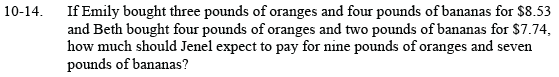Home > CCA2 > Chapter 10 > Lesson 10.1.1 > Problem10-14

10-14.

If Emily bought three pounds of oranges and four pounds of bananas for $8.53 and Beth bought four pounds of oranges and two pounds of bananas for$7.74, how much should Jenel expect to pay for nine pounds of oranges and seven pounds of bananas? Homework Help ✎Try writing a system of equations for the problem.

3(oranges) + 4(bananas) = $8.53 4(oranges) + 2(bananas) =$7.74

Solve the system of equations to determine the cost of 1 pound of oranges and 1 pound of bananas.
Use your answers to determine the cost of 9 pounds of oranges and 7 pounds of bananas.